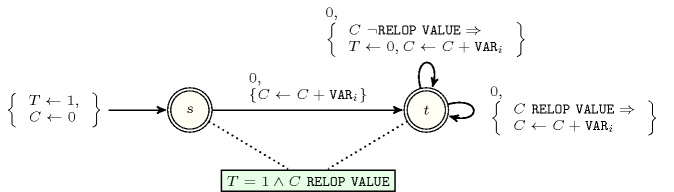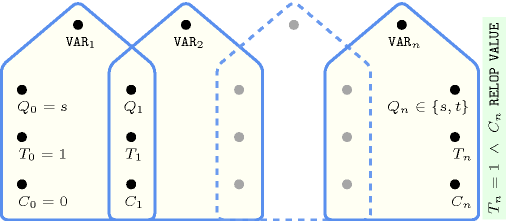## 5.33. arith_sliding

Origin

Used in the definition of some automaton

Constraint

$\mathrm{𝚊𝚛𝚒𝚝𝚑}_\mathrm{𝚜𝚕𝚒𝚍𝚒𝚗𝚐}\left(\mathrm{𝚅𝙰𝚁𝙸𝙰𝙱𝙻𝙴𝚂},\mathrm{𝚁𝙴𝙻𝙾𝙿},\mathrm{𝚅𝙰𝙻𝚄𝙴}\right)$

Arguments
 $\mathrm{𝚅𝙰𝚁𝙸𝙰𝙱𝙻𝙴𝚂}$ $\mathrm{𝚌𝚘𝚕𝚕𝚎𝚌𝚝𝚒𝚘𝚗}\left(\mathrm{𝚟𝚊𝚛}-\mathrm{𝚍𝚟𝚊𝚛}\right)$ $\mathrm{𝚁𝙴𝙻𝙾𝙿}$ $\mathrm{𝚊𝚝𝚘𝚖}$ $\mathrm{𝚅𝙰𝙻𝚄𝙴}$ $\mathrm{𝚒𝚗𝚝}$
Restrictions
 $\mathrm{𝚛𝚎𝚚𝚞𝚒𝚛𝚎𝚍}$$\left(\mathrm{𝚅𝙰𝚁𝙸𝙰𝙱𝙻𝙴𝚂},\mathrm{𝚟𝚊𝚛}\right)$ $\mathrm{𝚁𝙴𝙻𝙾𝙿}\in \left[=,\ne ,<,\ge ,>,\le \right]$
Purpose

Enforce for all sequences of variables ${\mathrm{𝚟𝚊𝚛}}_{1},{\mathrm{𝚟𝚊𝚛}}_{2},\cdots ,{\mathrm{𝚟𝚊𝚛}}_{i}$ $\left(1\le i\le |\mathrm{𝚅𝙰𝚁𝙸𝙰𝙱𝙻𝙴𝚂}|\right)$ of the $\mathrm{𝚅𝙰𝚁𝙸𝙰𝙱𝙻𝙴𝚂}$ collection to have $\left({\mathrm{𝚟𝚊𝚛}}_{1}+{\mathrm{𝚟𝚊𝚛}}_{2}+\cdots +{\mathrm{𝚟𝚊𝚛}}_{i}\right)\mathrm{𝚁𝙴𝙻𝙾𝙿}\mathrm{𝚅𝙰𝙻𝚄𝙴}$.

Example
$\left(〈0,0,1,2,0,0,-3〉,<,4\right)$

The $\mathrm{𝚊𝚛𝚒𝚝𝚑}_\mathrm{𝚜𝚕𝚒𝚍𝚒𝚗𝚐}$ constraint holds since all the following seven inequalities hold:

• $0<4$,

• $0+0<4$,

• $0+0+1<4$,

• $0+0+1+2<4$,

• $0+0+1+2+0<4$,

• $0+0+1+2+0+0<4$,

• $0+0+1+2+0+0-3<4$.

Typical
 $|\mathrm{𝚅𝙰𝚁𝙸𝙰𝙱𝙻𝙴𝚂}|>1$ $\mathrm{𝚁𝙴𝙻𝙾𝙿}\in \left[<,\ge ,>,\le \right]$
Arg. properties
• Contractible wrt. $\mathrm{𝚅𝙰𝚁𝙸𝙰𝙱𝙻𝙴𝚂}$ when $\mathrm{𝚁𝙴𝙻𝙾𝙿}\in \left[<,\le \right]$ and $\mathrm{𝚖𝚒𝚗𝚟𝚊𝚕}\left(\mathrm{𝚅𝙰𝚁𝙸𝙰𝙱𝙻𝙴𝚂}.\mathrm{𝚟𝚊𝚛}\right)\ge 0$.

• Suffix-contractible wrt. $\mathrm{𝚅𝙰𝚁𝙸𝙰𝙱𝙻𝙴𝚂}$.

Keywords
Arc input(s)

$\mathrm{𝚅𝙰𝚁𝙸𝙰𝙱𝙻𝙴𝚂}$

Arc generator
$\mathrm{𝑃𝐴𝑇𝐻}_\mathit{1}$$↦\mathrm{𝚌𝚘𝚕𝚕𝚎𝚌𝚝𝚒𝚘𝚗}$

Arc arity
$*$
Arc constraint(s)
$\mathrm{𝚊𝚛𝚒𝚝𝚑}$$\left(\mathrm{𝚌𝚘𝚕𝚕𝚎𝚌𝚝𝚒𝚘𝚗},\mathrm{𝚁𝙴𝙻𝙾𝙿},\mathrm{𝚅𝙰𝙻𝚄𝙴}\right)$
Graph property(ies)
$\mathrm{𝐍𝐀𝐑𝐂}$$=|\mathrm{𝚅𝙰𝚁𝙸𝙰𝙱𝙻𝙴𝚂}|$

Automaton

Figure 5.33.1 depicts the automaton associated with the $\mathrm{𝚊𝚛𝚒𝚝𝚑}_\mathrm{𝚜𝚕𝚒𝚍𝚒𝚗𝚐}$ constraint. To each item of the collection $\mathrm{𝚅𝙰𝚁𝙸𝙰𝙱𝙻𝙴𝚂}$ corresponds a signature variable ${S}_{i}$ that is equal to 0.

##### Figure 5.33.1. Automaton of the $\mathrm{𝚊𝚛𝚒𝚝𝚑}_\mathrm{𝚜𝚕𝚒𝚍𝚒𝚗𝚐}$ constraint ($T$ is initially set to 1 and reset to 0 as soon as one of the sliding constraints does not hold)##### Figure 5.33.2. Hypergraph of the reformulation corresponding to the automaton (with two counters) of the $\mathrm{𝚊𝚛𝚒𝚝𝚑}_\mathrm{𝚜𝚕𝚒𝚍𝚒𝚗𝚐}$ constraint (since all states of the automaton are accepting there is no restriction on the last variable ${Q}_{n}$)